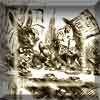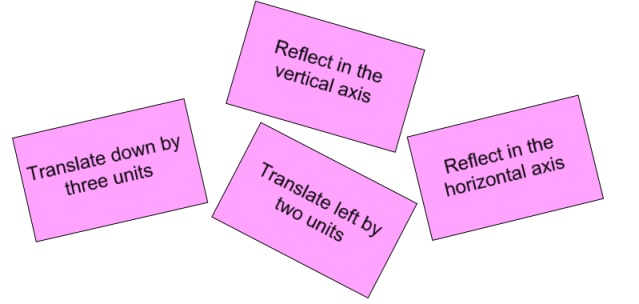#### You may also like### A Problem of Time

Consider a watch face which has identical hands and identical marks for the hours. It is opposite to a mirror. When is the time as read direct and in the mirror exactly the same between 6 and 7?### Tricircle

The centre of the larger circle is at the midpoint of one side of an equilateral triangle and the circle touches the other two sides of the triangle. A smaller circle touches the larger circle and two sides of the triangle. If the small circle has radius 1 unit find the radius of the larger circle.### Two Triangles in a Square

Given that ABCD is a square, M is the mid point of AD and CP is perpendicular to MB with P on MB, prove DP = DC.

# Surprising Transformations

##### Age 14 to 16 Challenge Level:

This problem follows on from Translating Lines and Reflecting Lines.

I took the graph $y=4x+7$ and performed the four transformations shown on the cards below.Unfortunately, I can't remember the order in which I carried out the four transformations, but I know that I ended up with the graph of $y=4x-2$.

Can you find an order in which I could have carried out the transformations?
There is more than one way of doing this - can you find them all?

Can you explain why different orders can lead to the same outcome?

What other lines could I have ended up with if I had performed the four transformations in a different order?